# Maharashtra Board 12th Maths Solutions Chapter 7 Linear Programming Miscellaneous Exercise 7

Balbharti 12th Maharashtra State Board Maths Solutions Book Pdf Chapter 7 Linear Programming Miscellaneous Exercise 7 Questions and Answers.

## Maharashtra State Board 12th Maths Solutions Chapter 7 Linear Programming Miscellaneous Exercise 7

I) Select the appropriate alternatives for each of the following :
Question 1.
The value of objective function is maximum under linear constraints _______.
(A) at the centre of the feasible region
(B) at (0, 0)
(C) at a vertex of the feasible region
(D) the vertex which is of maximum distance from (0, 0)
Solution:
(C) at a vertex of the feasible region

Question 2.
Which of the following is correct _______.
(A) every L.P.P. has an optimal solution
(B) a L.P.P. has unique optimal solution
(C) if L.P.P. has two optimal solutions then it has an infinite number of optimal solutions
(D) the set of all feasible solutions of L.P.P. may not be a convex set
Solution:
(C) if L.P.P. has two optimal solutions then it has an infinite number of optimal solutions

Question 3.
Objective function of L.P.P. is _______.
(A) a constraint
(B) a function to be maximized or minimized
(C) a relation between the decision variables
(D) equation of a straight line
Solution:
(B) a function to be maximized or minimized

Question 4.
The maximum value of z = 5x + 3y subjected to the constraints 3x + 5y ≤ 15, 5x + 2y ≤ 10, x, y≥ 0 is _______.
(A) 235
(B) $$\frac{235}{9}$$
(C) $$\frac{235}{19}$$
(D) $$\frac{235}{3}$$
Solution:
(C) $$\frac{235}{19}$$Question 5.
The maximum value of z = 10x + 6y subjected to the constraints 3x + y ≤ 12, 2x + 5y ≤ 34, x ≥ 0, y≥ 0. _______.
(A) 56
(B) 65
(C) 55
(D) 66
Solution:
(A) 56

Question 6.
The point at which the maximum value of x + y subject to the constraints x + 2y ≤ 70, 2x + y ≤ 95, x ≥ 0, y ≥ 0 is obtained at _______.
(A) (30, 25)
(B) (20, 35)
(C) (35, 20)
(D) (40, 15)
Solution:
(D) (40, 15)

Question 7.
Of all the points of the feasible region, the optimal value of obtained at the point lies _______.
(A) inside the feasible region
(B) at the boundary of the feasible region
(C) at the vertex of the feasible region
(D) outside the feasible region
Solution:
(C) at the vertex of the feasible regionQuestion 8.
The feasible region is the set of points that satisfy _______.
(A) the objective function
(B) all of the given constraints
(C) some of the given constraints
(D) only one constraint
Solution:
(B) all of the given constraints

Question 9.
Solution of L.P.P. to minimize z = 2x + 3y such that x ≥ 0, y ≥ 0, 1 ≤ x + 2y ≤ 10 is _______.
(A) x = 0, y = $$\frac{1}{2}$$
(B) x = $$\frac{1}{2}$$, y = 0
(C) x = 1, y = 2
(D) x = $$\frac{1}{2}$$, y = $$\frac{1}{2}$$
Solution:
(A) x = 0, y = $$\frac{1}{2}$$

Question 10.
The corner points of the feasible solution given by the inequation x + y ≤ 4, 2x + y ≤ 7, x ≥ 0, y ≥ 0 are _______.
(A) (0, 0), (4, 0), (7, 1), (0, 4)
(B) (0, 0), ($$\frac{7}{2}$$, 0), (3, 1), (0, 4)
(C) (0, 0), ($$\frac{7}{2}$$, 0), (3, 1), (0, 7)
(D) (0, 0), (4, 0), (3, 1), (0, 7)
Solution:
(B) (0, 0), ($$\frac{7}{2}$$, 0), (3, 1), (0, 4)

Question 11.
The corner points of the feasible solution are (0, 0), (2, 0), ($$\frac{12}{7}$$, $$\frac{3}{7}$$), (0, 1). Then z = 7x + y is maximum at _______.
(A) (0, 0)
(B) (2, 0)
(C) ($$\frac{12}{7}$$, $$\frac{3}{7}$$)
(D) (0, 1)
Solution:
(B) (2, 0)Question 12.
If the corner points of the feasible solution are (0, 0), (3, 0), (2, 1) and (0, $$\frac{7}{3}$$ ), the maximum value of z = 4x + 5y is _______.
(A) 12
(B) 13
(C) 35
(D) 0
Solution:
(B) 13

Question 13.
If the corner points of the feasible solution are (0, 10), (2, 2) and (4, 0) then the point of minimum z = 3x + 2y is _______.
(A) (2, 2)
(B) (0, 10)
(C) (4, 0)
(D) (3 ,4)
Solution:
(A) (2, 2)

Question 14.
The half plane represented by 3x + 2y < 8 contains the point _______.
(A) (1, $$\frac{5}{2}$$)
(B) (2, 1)
(C) (0, 0)
(D) (5, 1)
Solution:
(C) (0, 0)Question 15.
The half plane represented by 4x + 3y > 14 contains the point _______.
(A) (0, 0)
(B) (2, 2)
(C) (3, 4)
(D) (1, 1)
Solution:
(C) (3, 4)

II) Solve the following :
Question 1.
Solve each of the following inequations graphically using X Y plane.
(i) 4x – 18 ≥ 0
Solution:
Consider the line whose equation is 4x – 18 ≥ 0 i.e. x = $$\frac{18}{4}=\frac{9}{2}$$ = 4.5
This represents a line parallel to Y-axis passing3through the point (4.5, 0)
Draw the line x = 4.5
To find the solution set we have to check the position of the origin (0, 0).
When x = 0, 4x – 18 = 4 × 0 – 18 = -18 > 0
∴ the coordinates of the origin does not satisfy thegiven inequality.
∴ the solution set consists of the line x = 4.5 and the non-origin side of the line which is shaded in the graph.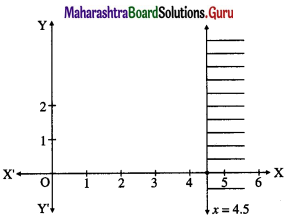(ii) -11x – 55 ≤ 0
Solution:
Consider the line whose equation is -11x – 55 ≤ 0 i.e. x = -5
This represents a line parallel to Y-axis passing3through the point (-5, 0)
Draw the line x = – 5
To find the solution set we have to check the position of the origin (0, 0).
When x = 0, -11x – 55 = – 11(0) – 55 = -55 > 0
∴ the coordinates of the origin does not satisfy thegiven inequality.
∴ the solution set consists of the line x = -5 and the non-origin side of the line which is shaded in the graph.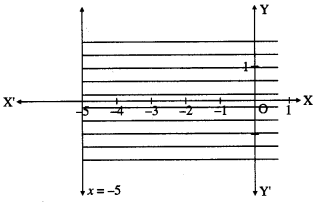(iii) 5y – 12 ≥ 0
Solution:
Consider the line whose equation is 5y – 12 ≥ 0 i.e. y = $$\frac{12}{5}$$
This represents a line parallel to X-axis passing through the point (o, $$\frac{12}{5}$$)
Draw the line y = $$\frac{12}{5}$$
To find the solution set, we have to check the position of the origin (0, 0).
When y = 0, 5y – 12 = 5(0) – 12 = -12 > 0
∴ the coordinates of the origin does not satisfy the given inequality.
∴ the solution set consists of the line y = $$\frac{12}{5}$$ and the non-origin side of the line which is shaded in the graph.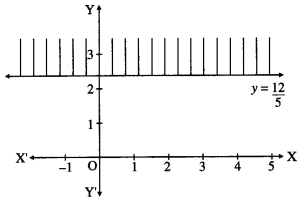(iv) y ≤ -3.5
Solution:
Consider the line whose equation is y ≤ – 3.5 i.e. y = – 3.5
This represents a line parallel to X-axis passing3through the point (0, -3.5)
Draw the line y = – 3.5
To find the solution set, we have to check the position of the origin (0, 0).
∴ the coordinates of the origin does not satisfy the given inequality.
∴ the solution set consists of the line y = – 3.5 and the non-origin side of the line which is shaded in the graph.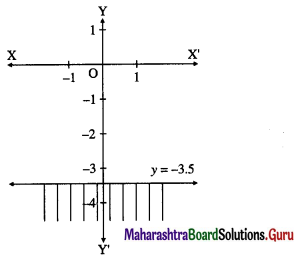Question 2.
Sketch the graph of each of following inequations in XOY co-ordinate system.
(i) x ≥ 5y
Solution: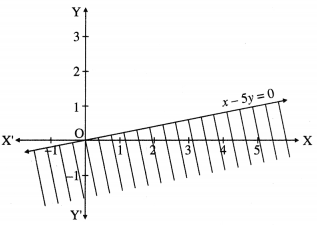(ii) x + y≤ 0
Solution: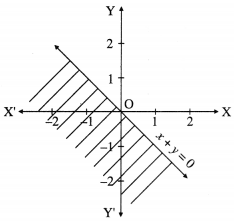(iii) 2y – 5x ≥ 0
Solution: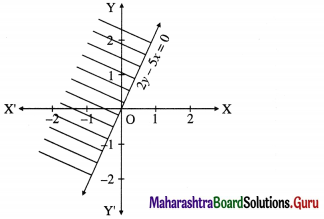(iv) |x + 5| ≤ y
Solution:
|x + 5| ≤ y
∴ -y ≤ x + 5 ≤ y
∴ -y ≤ x + 5 and x + 5 ≤ y
∴ x + y ≥ -5 and x – y ≤ -5
First we draw the lines AB and AC whose equations are
x + y= -5 and x – y = -5 respectively.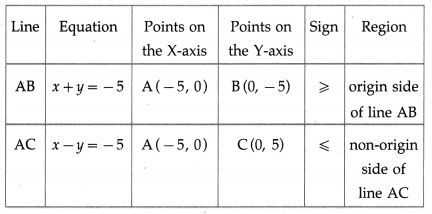The graph of |x + 5| ≤ y is as below: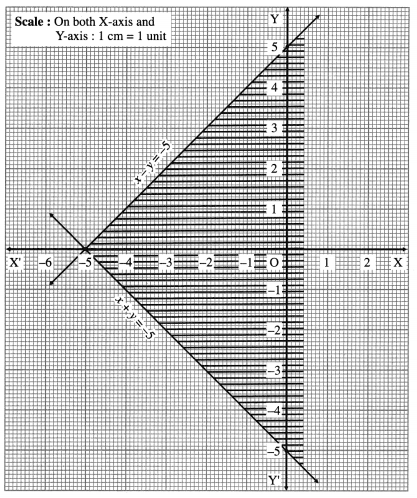Question 3.
Find graphical solution for each of the following system of linear inequation.
(i) 2x + y ≥ 2, x – y ≤ 1
Solution:
First we draw the lines AB and AC whose equations are 2x + y = 2 and x – y = 1 respectively.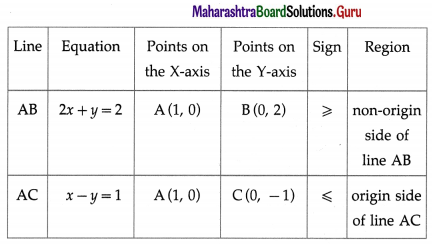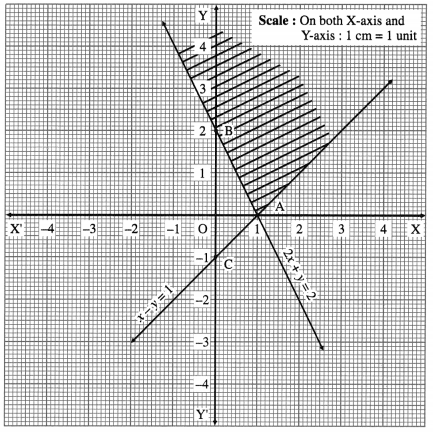The solution set of the given system of inequalities is shaded in the graph.

(ii) x + 2y ≥ 4, 2x – y ≤ 6
Solution: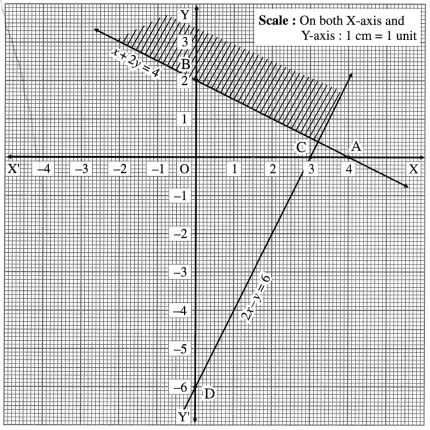(iii) 3x + 4y ≤ 12, x – 2y ≥ 2, y ≥ -1
Solution:
First we draw the lines AB, CD and ED whose equations are 3x + 4y = 12, x – 2y = 2 and y = -1 respectively.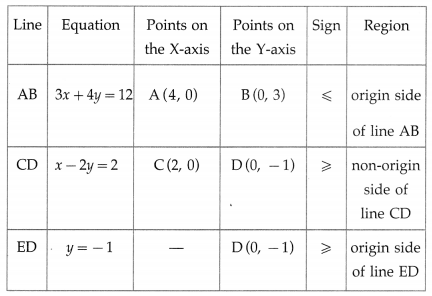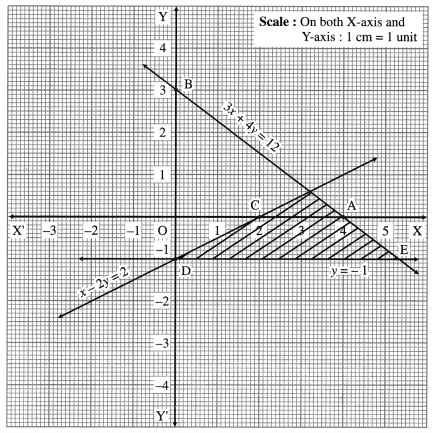The solution set of given system of inequation is shaded in the graph.

Question 4.
Find feasible solution for each of the following system of linear inequations graphically.
(i) 2x + 3y ≤ 12, 2x + y ≤ 8, x ≥ 0, y ≥ 0
Solution: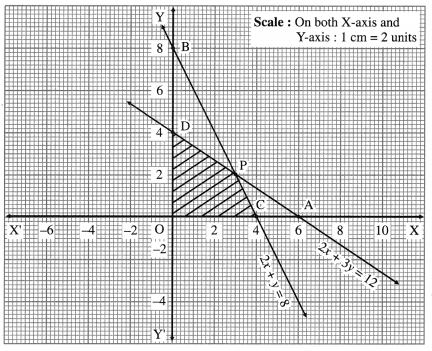The feasible solution is OCPBO.

(ii) 3x + 4y ≥ 12, 4x + 7y ≤ 28, x ≥ 0, y ≥ 0
Solution: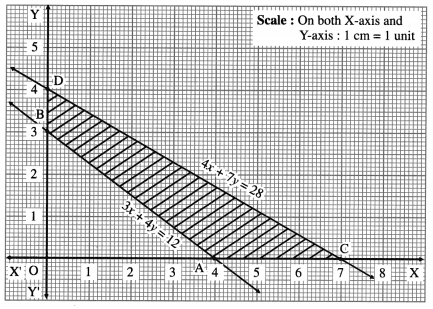The feasible solution is ACDBA.Question 5.
Solve each of the following L.P.P.
(i) Maximize z = 5x1 + 6x2 subject to 2x1 + 3x2 ≤ 18, 2x1 + x2 ≤ 12, x1 ≥ 0, x2 ≥ 0
Solution:
First we draw the lines AB and CD whose equations are 2x1 + 3x2 = 18 and 2x1 + x2 = 12 respectively.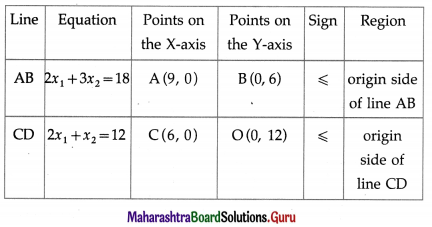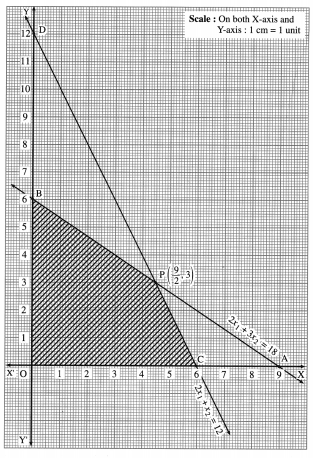The feasible region is OCPBO which is shaded in the graph. The vertices of the feasible region are O(0, 0), C(6, 0), P and B (0,6).
P is the point of intersection of the lines
2x1 + 3x2 = 18 ….(1)
and 2x1 + x2 = 12
On subtracting, we get
2x2 = 6 ∴ x2 = 3
Substituting x2 = 3 in (2), we get
2x1 + 3 = 12 ∴ x2 = 9
∴ P is ($$\frac{9}{2}$$, 3)
The values of objective function z = 5x1 + 6x2 at these vertices are
z(O) = 5(0) + 6(0) = 0 + 0 = 0
z(C) = 5(6) + 6(0) = 30 + 0 = 30
z(P) = 5($$\frac{9}{2}$$) + 6(3) = $$\frac{45}{2}$$ + 18 = $$\frac{45+36}{2}=\frac{81}{2}$$ = 40.5
z(B) = 5(0) + 6(3) = 0 + 18 = 18
Maximum value of z is 40.5 when x1 = 9/2, y = 3.

(ii) Maximize z = 4x + 2y subject to 3x + y ≥ 27, x + y ≥ 21
Question is modified.
Maximize z = 4x + 2y subject to 3x + y ≤ 27, x + y ≤ 21, x ≥ 0, y ≥ 0
Solution:
First we draw the lines AB and CD whose equations are 3x + y = 27 and x + y = 21 respectively.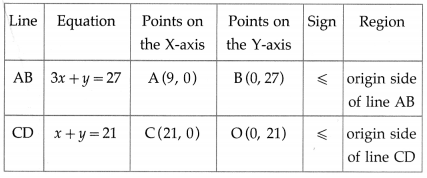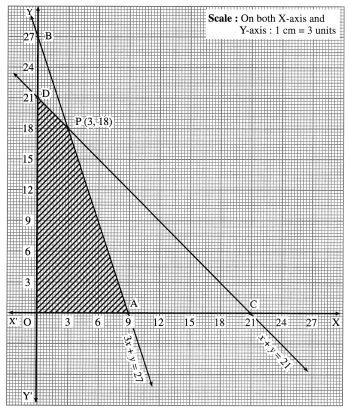The feasible region is OAPDO which is shaded region in the graph. The vertices of the feasible region are 0(0, 0), A (9, 0), P and D(0, 21). P is the point of intersection of lines
3x + y = 27 … (1)
and x + y = 21 … (2)
On substracting, we get 2x = 6 ∴ x = 3
Substituting x = 3 in equation (1), we get
9 + y = 27 ∴ y = 18
∴ P = (3, 18)
The values of the objective function z = 4x + 2y at these vertices are
z(O) = 4(0) + 2(0) = 0 + 0 = 0
z(a) = 4(9) + 2(0) = 36 + 0 = 36
z(P) = 4(3) + 2(18) = 12 + 36 = 48
z (D) = 4(0) + 2(21) = 0 + 42 = 42
∴ 2 has minimum value 48 when x = 3, y = 18.

(iii) Maximize z = 6x + 10y subject to 3x + 5y ≤ 10, 5x + 3y ≤ 15, x ≥ 0, y ≥ 0
Solution:
First we draw the lines AB and CD whose equations are 3x + 5y = 10 and 5x + 3y = 15 respectively.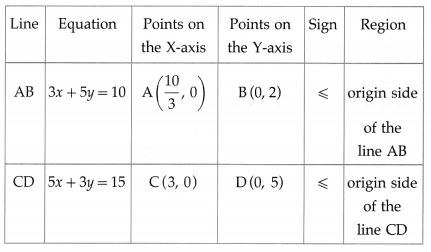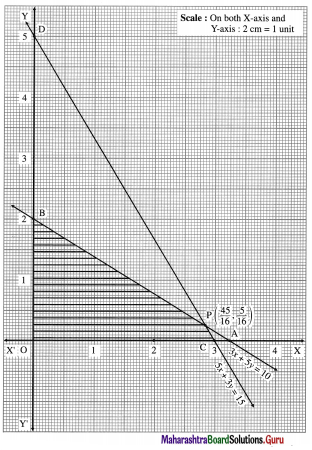The feasible region is OCPBD which is shaded in the graph.
The vertices of the feasible region are 0(0, 0), C(3, 0), P and B (0, 2).
P is the point of intersection of the lines
3x + 5y = 10 … (1)
and 5x + 3y = 15 … (2)
Multiplying equation (1) by 5 and equation (2) by 3, we get
15x + 25y = 50
15x + 9y = 45
On subtracting, we get
16y = 5 ∴ y = $$\frac{5}{16}$$
Substituting y = $$\frac{5}{16}$$ in equation (1), we get
3x + $$\frac{25}{16}$$ = 10 ∴ 3x = 10 – $$\frac{25}{16}=\frac{135}{16}$$
∴ x = $$\frac{45}{16}$$ ∴ P ≡ $$\left(\frac{45}{16}, \frac{5}{16}\right)$$
The values of objective function z = 6x + 10y at these vertices are
z(O) = 6(0) + 10(0) = 0 + 0 = 0
z(C) = 6(3) + 10(0) = 18 + 0 = 18
z(P) = 6$$\left(\frac{45}{16}\right)$$ + 10$$\left(\frac{5}{10}\right)$$ = $$\frac{270}{16}+\frac{50}{16}=\frac{320}{16}$$ = 20
z(B) = 6(0) + 10(2) = 0 + 20 = 20
The maximum value of z is 20 at P$$\left(\frac{45}{16}, \frac{5}{16}\right)$$ and B (0, 2) two consecutive vertices.
∴ z has maximum value 20 at each point of line segment PB where B is (0, 2) and P is $$\left(\frac{45}{16}, \frac{5}{16}\right)$$.
Hence, there are infinite number of optimum solutions.(iv) Maximize z = 2x + 3y subject to x – y ≥ 3, x ≥ 0, y ≥ 0
Solution:
First we draw the lines AB whose equation is x – y = 3.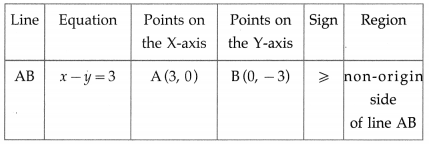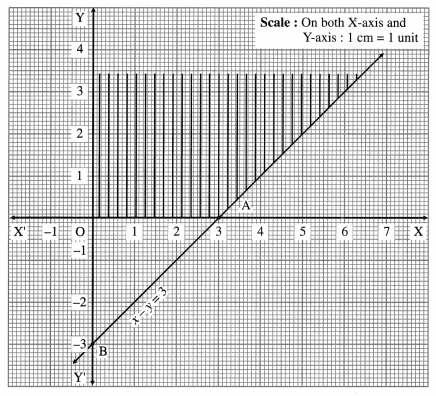The feasible region is shaded which is unbounded.
Therefore, the value of objective function can be in- j creased indefinitely. Hence, this LPP has unbounded solution.

Question 6.
Solve each of the following L.P.P.
(i) Maximize z = 4x1 + 3x2 subject to 3x1 + x2 ≤ 15, 3x1 + 4x2 ≤ 24, x1 ≥ 0, x2 ≥ 0
Solution:
We first draw the lines AB and CD whose equations are 3x1 + x2 = 15 and 3x1 + 4x2 = 24 respectively.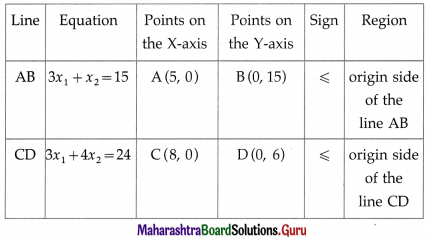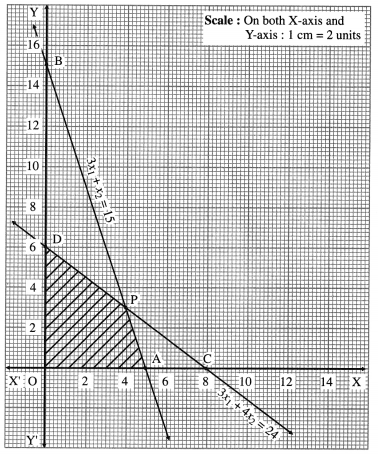The feasible region is OAPDO which is shaded in the graph.
The Vertices of the feasible region are 0(0, 0), A(5, 0), P and D(0, 6).
P is the point of intersection of lines.
3x1 + 4x2 = 24 … (1)
and 3x1 + x2 = 15 … (2)
On subtracting, we get
3x2 = 9 ∴ x2 = 3
Substituting x2 = 3 in (2), we get
3x1 + 3 = 15
∴ 3x1 = 12 ∴ x1 = 4 ∴ P is (4, 3)
The values of objective function z = 4x1 + 3x2 at these vertices are
z(O) = 4(0) + 3(0) = 0 + 0 = 0
z(a) = 4(5) + 3(0) = 20 + 0 = 20
z(P) = 4(4) + 3(3) = 16 + 9 = 25
z(D) = 4(0) + 3(6) = 0 + 18 = 18
∴ z has maximum value 25 when x = 4 and y = 3.

(ii) Maximize z = 60x + 50y subject to x + 2y ≤ 40, 3x + 2y ≤ 60, x ≥ 0, y ≥ 0
Solution:
We first draw the lines AB and CD whose equations are x + 2y = 40 and 3x + 2y = 60 respectively.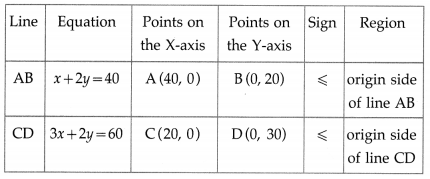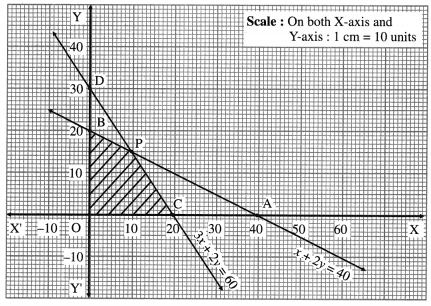The feasible region is OCPBO which is shaded in the graph.
The vertices of the feasible region are O (0, 0), C (20, 0), P and B (0, 20).
P is the point of intersection of the lines.
3x + 2y = 60 … (1)
and x + 2y = 40 … (2)
On subtracting, we get
2x = 20 ∴ x = 10
Substituting x = 10 in (2), we get
10 + 2y = 40
∴ 2y = 30 ∴ y = 15 ∴ P is (10, 15)
The values of the objective function z = 60x + 50y at these vertices are
z(O) = 60(0) + 50(0) = 0 + 0 = 0
z(C) = 60(20) + 50(0) = 1200 + 0 = 1200
z(P) = 60(10) + 50(15) = 600 + 750 = 1350
z(B) = 60(0) + 50(20) = 0 + 1000 = 1000 .
∴ z has maximum value 1350 at x = 10, y = 15.(iii) Maximize z = 4x + 2y subject to 3x + y ≥ 27, x + y ≥ 21, x + 2y ≥ 30; x ≥ 0, y ≥ 0
Solution:
We first draw the lines AB, CD and EF whose equations are 3x + y = 27, x + y = 21, x + 2y = 30 respectively.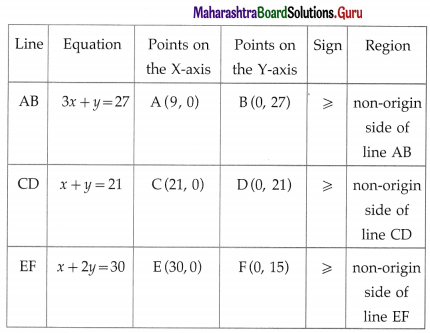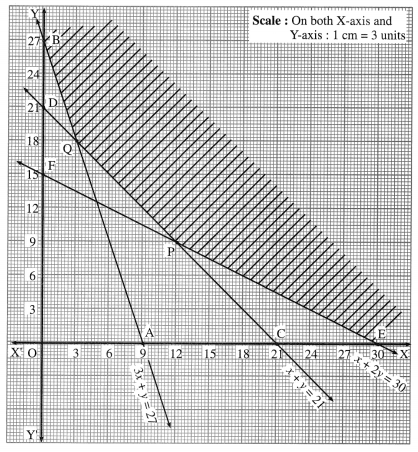The feasible region is XEPQBY which is shaded in the graph.
The vertices of the feasible region are E (30,0), P, Q and B (0,27).
P is the point of intersection of the lines
x + 2y = 30 … (1)
and x + y = 21 … (2)
On subtracting, we get
y = 9
Substituting y = 9 in (2), we get
x + 9 = 21 ∴ x = 12
∴ P is (12, 9)
Q is the point of intersection of the lines
x + y = 21 … (2)
and 3x + y = 27 … (3)
On subtracting, we get
2x = 6 ∴ x = 3
Substituting x = 3 in (2), we get
3 + y = 21 ∴ y = 18
∴ Q is (3, 18).
The values of the objective function z = 4x + 2y at these vertices are
z(E) = 4(30) + 2(0) = 120 + 0 = 120
z(P) = 4(12) + 2(9) = 48 + 18 = 66
z(Q) = 4(3) + 2(18) = 12 + 36 = 48
z(B) = 4(0) + 2(27) = 0 + 54 = 54
∴ z has minimum value 48, when x = 3 and y = 18.

Question 7.
A carpenter makes chairs and tables. Profits are ₹140/- per chair and ₹ 210/- per table. Both products are processed on three machines : Assembling, Finishing and Polishing. The time required for each product in hours and availability of each machine is given by following table: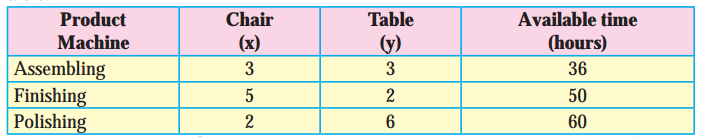Formulate the above problem as L.P.P. Solve it graphically to get maximum profit.
Solution:
Let the number of chairs and tables made by the carpenter be x and y respectively.
The profits are ₹ 140 per chair and ₹ 210 per table.
∴ total profit z = ₹ (140x + 210y) This is the objective function which is to be maximized.
The constraints are as per the following table :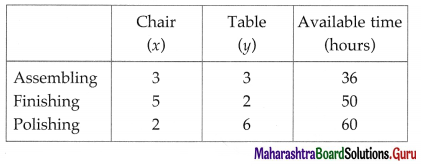From the table, the constraints are
3x + 3y ≤ 36, 5x + 2y ≤ 50, 2x + 6y ≤ 60.
The number of chairs and tables cannot be negative.
∴ x ≥ 0, y ≥ 0
Hence, the mathematical formulation of given LPP is :
Maximize z = 140x + 210y, subject to
3x + 3y ≤ 36, 5x + 2y ≤ 50, 2x + 6y ≤ 60, x ≥ 0, y ≥ 0.
We first draw the lines AB, CD and EF whose equations are 3x + 3y = 36, 5x + 2y = 50 and 2x + 6y = 60 respectively.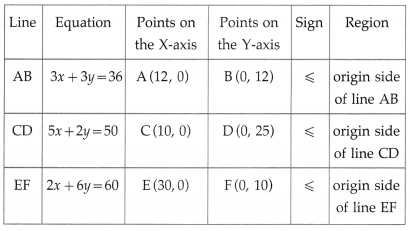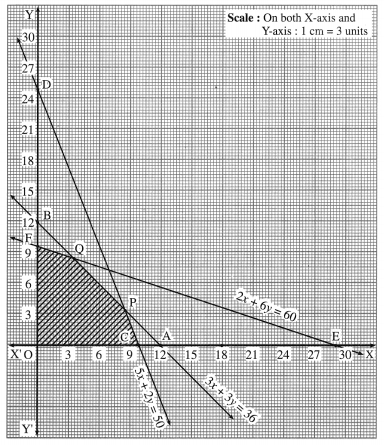The feasible region is OCPQFO which is shaded in the graph.
The vertices of the feasible region are O (0, 0), C (10, 0), P, Q and F (0, 10).
P is the point of intersection of the lines
5x + 2y = 50 … (1)
and 3x + 3y = 36 … (2)
Multiplying equation (1) by 3 and equation (2) by 2, we get
15x + 6y = 150
6x + 6y = 72
On subtracting, we get 26
9x = 78 ∴ x = $$\frac{26}{3}$$
Substituting x = $$\frac{26}{3}$$ in (2), we get
3$$\left(\frac{26}{3}\right)$$ + 3y = 36
3y = 1o y = $$\frac{10}{3}$$
Q is the point of intersection of the lines
3x + 3y = 36 … (2)
and 2x + 6y = 60 … (3)
Multiplying equation (2) by 2, we get
6x + 6 y = 72
Subtracting equation (3) from this equation, we get
4x = 12 ∴ x = 3
Substituting x = 3 in (2), we get
3(3) + 3y = 36
∴ 3y = 27 ∴ y = 9
∴ Q is (3, 9).
Hence, the vertices of the feasible region are O (0, 0),
C(10, 0), P$$\left(\frac{26}{3}, \frac{10}{3}\right)$$, Q(3, 9) and F(0, 10).
The values of the objective function z = 140x + 210y at these vertices are
z(O) = 140(0) + 210(0) = 0 + 0 = 0
z(C) = 140 (10) + 210(0) = 1400 + 0 = 1400
z(P) = 140$$\left(\frac{26}{3}\right)$$ + 210$$\left(\frac{10}{3}\right)$$ = $$\frac{3640+2100}{3}=\frac{5740}{3}$$ = 1913.33
z(Q) = 140(3) + 210(9) = 420 + 1890 = 2310
z(F) = 140(0) + 210(10) = 0 + 2100 = 2100
∴ z has maximum value 2310 when x = 3 and y = 9 Hence, the carpenter should make 3 chairs and 9 tables to get the maximum profit of ₹ 2310.Question 8.
A company manufactures bicycles and tricycles, each of which must be processed through two machines A and B. Maximum availability of Machine A and B is respectively 120 and 180 hours. Manufacturing a bicycle requires 6 hours on Machine A and 3 hours on Machine B. Manufacturing a tricycles requires 4 hours on Machine A and 10 hours on Machine B. If profits are ₹180/- for a bicycle and ₹220/- for a tricycle. Determine the number of bicycles and tricycles that should be manufactured in order to maximize the profit.
Solution:
Let x bicycles and y tricycles are to be manu¬factured. Then the total profit is z = ₹ (180x + 220y)
This is a linear function which is to be maximized. Hence, it is the objective function. The constraints are as per the following table :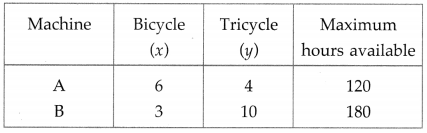From the table, the constraints are
6x + 4y ≤ 120, 3x +10y ≤ 180
Also, the number of bicycles and tricycles cannot be i negative.
∴ x ≥ 0, y ≥ 0.
Hence, the mathematical formulation of given LPP is :
Maximize z = 180x + 220y, subject to
6x + 4y ≤ 120, 3x + 10y ≤ 180, x ≥ 0, y ≥ 0.
First we draw the lines AB and CD whose equations are 6x + 4y = 120 and 3x + 10y = 180 respectively.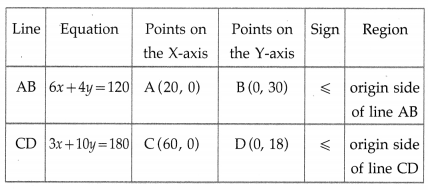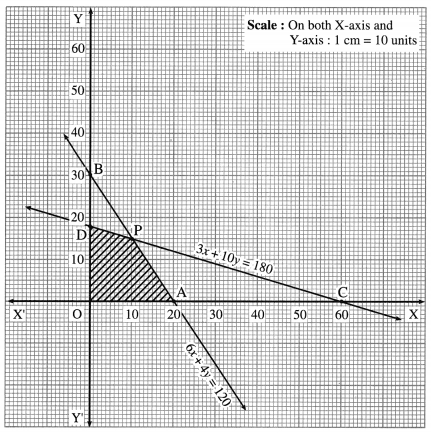The feasible region is OAPDO which is shaded in the graph.
The vertices of the feasible region are O(0, 0), A(20, 0) P and D(0, 18).
P is the point of intersection of the lines
3x + 10y = 180 … (1)
and 6x + 4y = 120 … (2)
Multiplying equation (1) by 2, we get
6x + 20y = 360
Subtracting equation (2) from this equation, we get
16y = 240 ∴ y = 15
∴ from (1), 3x + 10(15) = 180
∴ 3x = 30 ∴ x = 10
∴ P = (10, 15)
The values of the objective function z = 180x + 220y at these vertices are
z(O) = 180(0) + 220(0) = 0 + 0 = 0
z(a) = 180(20) + 220(0) = 3600 + 0 = 3600
z(P) = 180(10) + 220(15) = 1800 + 3300 = 5100
z(D) = 180(0) +220(18) = 3960
∴ the maximum value of z is 5100 at the point (10, 15).
Hence, 10 bicycles and 15 tricycles should be manufactured in order to have the maximum profit of ₹ 5100.

Question 9.
A factory produced two types of chemicals A and B. The following table gives the units of ingredients P and Q (per kg) of chemicals A and B as well as minimum requirements of P and Q and also cost per kg. chemicals A and B :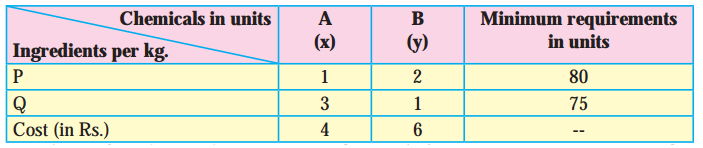Find the number of units of chemicals A and B should be produced so as to minimize the cost.
Solution:
Let the factory produce x units of chemical A and y units of chemical B. Then the total cost is z = ₹ (4x + 6y). This is the objective function which is to be minimized.
From the given table, the constraints are
x + 2y ≥ 80, 3x + y ≥ 75.
Also, the number of units x and y of chemicals A and B cannot be negative.
∴ x ≥ 0, y ≥ 0.
∴ the mathematical formulation of given LPP is
Minimize z = 4x + 6y, subject to
x + 2y ≥ 80, 3x + y ≥ 75, x ≥ 0, y ≥ 0.
First we draw the lines AB and CD whose equations are x + 2y = 80 and 3x + y = 75 respectively.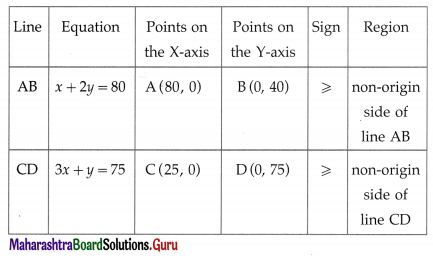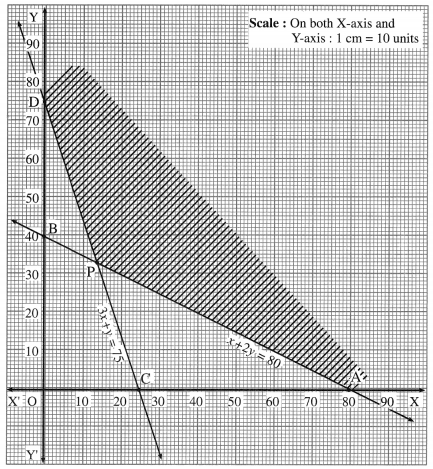The feasible region is shaded in the graph.
The vertices of the feasible region are A (80, 0), P and D (0, 75).
P is the point of intersection of the lines
x + 2y = 80 … (1)
and 3x + y = 75 … (2)
Multiplying equation (2) by 2, we get
6x + 2 y = 150
Subtracting equation (1) from this equation, we get
5x = 70 ∴ x = 14
∴ from (2), 3(14) + y = 75
∴ 42 + y = 75 ∴ y = 33
∴ P = (14, 33)
The values of the objective function z = 4x + 6y at these vertices are
z(a) = 4(80)+ 6(0) =320 + 0 = 320
z(P) = 4(14)+ 6(33) = 56+ 198 = 254
z(D) = 4(0) + 6(75) = 0 + 450 = 450
∴ the minimum value of z is 254 at the point (14, 33).
Hence, 14 units of chemical A and 33 units of chemical B are to be produced in order to have the j minimum cost of ₹ 254.Question 10.
A company produces mixers and food processors. Profit on selling one mixer and one food processor is ₹ 2,000/- and ₹ 3,000/- respectively. Both the products are processed through three Machines A, B, C. The time required in hours by each product and total time available in hours per week on each machine are as follows :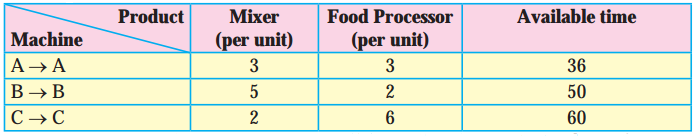How many mixers and food processors should be produced to maximize the profit?
Solution:
Let the company produce x mixers and y food processors.
Then the total profit is z = ₹ (2000x + 3000y)
This is the objective function which is to be maximized. From the given table in the problem, the constraints are 3x + 3y ≤ 36, 5x + 2y ≤ 50, 2x + 6y ≤ 60
Also, the number of mixers and food processors cannot be negative,
∴ x ≥ 0, y ≥ 0.
∴ the mathematical formulation of given LPP is
Maximize z = 2000x + 3000y, subject to 3x + 3y ≤ 36, 5x + 2y ≤ 50, 2x + 6y ≤60, x ≥ 0, y ≥ 0.
First we draw the lines AB, CD and EF whose equations are 3x + 3y = 36, 5x + 2y = 50 and 2x + 6y = 60 respectively.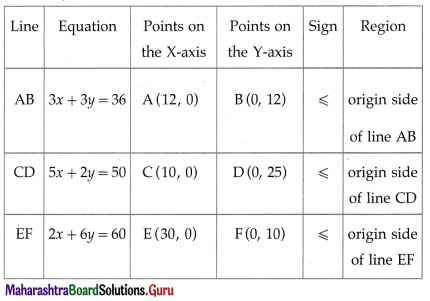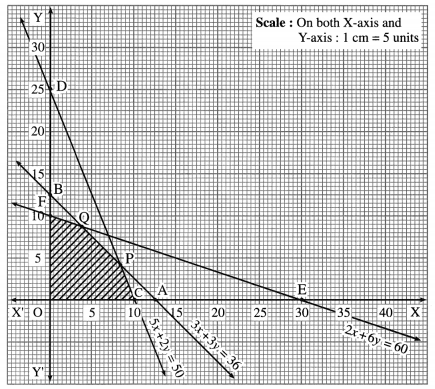The feasible region is OCPQFO which is shaded in the graph.
The vertices of the feasible region are O(0, 0), C(10, 0), P, Q and F(0,10).
P is the point of intersection of the lines
3x + 3y = 36 … (1)
and 5x + 2y = 50 … (2)
Multiplying equation (1) by 2 and equation (2) by 3, we get
6x + 6y = 72
15x + 6y = 150
On subtracting, we get
9x = 78
∴ x = $$\frac{26}{3}$$
∴ from (1), 3$$\left(\frac{26}{3}\right)$$ + 3y = 36
∴ 3y = 10
∴ y = $$\frac{10}{3}$$
∴ P = $$\left(\frac{26}{3}, \frac{10}{3}\right)$$
Q is the point of intersection of the lines
3x + 3y = 36 … (1)
and 2x + 6y = 60 … (3)
Multiplying equation (1) by 2, we get
6x + 6y = 72
Subtracting equation (3), from this equation, we get
4x = 12
∴ x = 3
∴ from (1), 3(3) + 3y = 36
∴ 3y = 27
∴ y = 9
∴ Q = (3, 9)
The values of the objective function z = 2000x + 3000y at these vertices are
z(O) = 2000(0) + 3000(0) = 0 + 0 = 0
z(C) = 2000(10) + 3000(0) = 20000 + 0 = 20000
z(P) = 2000$$\left(\frac{26}{3}\right)$$ + 3000$$\left(\frac{10}{3}\right)$$ = $$\frac{52000}{3}+\frac{30000}{3}=\frac{82000}{3}$$
z(Q) = 2000(3) + 3000(9) = 6000 + 27000 = 33000
z(F) = 2000(0) + 3000(10) = 30000 + 0 = 30000
∴ the maximum value of z is 33000 at the point (3, 9).
Hence, 3 mixers and 9 food processors should be produced in order to get the maximum profit of ₹ 33,000.

Question 11.
A chemical company produces a chemical containing three basic elements A, B, C so that it has at least 16 liters of A, 24 liters of B and 18 liters of C. This chemical is made by mixing two compounds I and II. Each unit of compound I has 4 liters of A, 12 liters of B, 2 liters of C. Each unit of compound II has 2 liters of A, 2 liters of B and 6 liters of C. The cost per unit of compound I is ₹ 800/- and that of compound II is ₹ 640/-. Formulate the problem as L.P.P. and solve it to minimize the cost.
Solution:
Let the company buy x units of compound I and y units of compound II.
Then the total cost is z = ₹(800x + 640y).
This is the objective function which is to be minimized.
The constraints are as per the following table :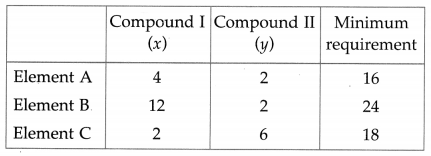From the table, the constraints are
4x + 2y ≥ 16, 12x + 2y ≥ 24, 2x + 6y ≥ 18.
Also, the number of units of compound I and compound II cannot be negative.
∴ x ≥ 0, y ≥ 0.
∴ the mathematical formulation of given LPP is
Minimize z = 800x + 640y, subject to 4x + 2y ≥ 16, 12x + 2y ≥ 24, 2x + 6y ≥ 18, x ≥ 0, y ≥ 0.
First we draw the lines AB, CD and EF whose equations are 4x + 2y = 16, 12x + 2y = 24 and 2x + 6y = 18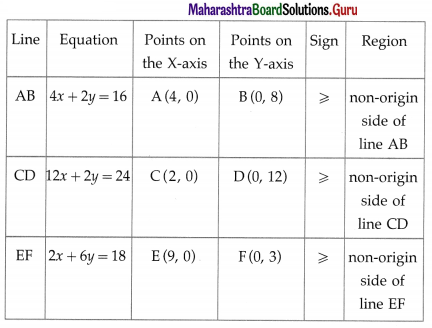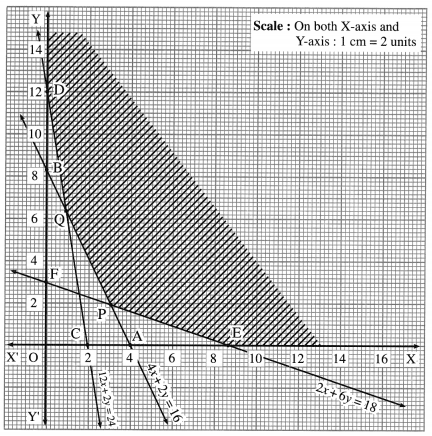The feasible region is shaded in the graph.
The vertices of the feasible region are E(9, 0), P, Q, and D(0, 12).
P is the point of intersection of the lines
2x + 6y = 18 … (1)
and 4x + 2y = 16 … (2)
Multiplying equation (1) by 2, we get 4x + 12y = 36
Subtracting equation (2) from this equation, we get
10y = 20
∴ y = 2
∴ from (1), 2x + 6(2) = 18
∴ 2x = 6
∴ x = 3
∴ P = (3, 2)
Q is the point of intersection of the lines
12x + 2y = 24 … (3)
and 4x + 2y = 16 … (2)
On subtracting, we get
8x = 8 ∴ x = 1
∴ from (2), 4(1) + 2y = 16
∴ 2y = 12 ∴ y = 6
∴ Q = (1, 6)
The values of the objective function z = 800x + 640y at these vertices are
z(E) = 800(9)+ 640(0) =7200 + 0 = 7200
z(P) = 800(3) + 640(2) = 2400 + 1280 = 3680
z(Q) = 800(1) + 640(6) =800 + 3840 =4640
z(D) = 800(0) + 640(12) = 0 + 7680 = 7680
∴ the minimum value of z is 3680 at the point (3, 2).
Hence, the company should buy 3 units of compound I and 2 units of compound II to have the minimum cost of ₹ 3680.Question 12.
A person makes two types of gift items A and B requires the services of a cutter and a finisher. Gift item A requires 4 hours of cutter’s time and 2 hours of finisher’s time. B requires 2 hours of cutter’s time and 4 hours of finisher’s time. The cutter and finisher have 208 hours and 152 hours available times respectively every month. The profit of one gift item of type A is ₹ 75/- and on gift item B is ₹ 125/-. Assuming that the person can sell all the gift items produced, determine how many gift items of each type should he make every month to obtain the best returns?
Solution:
Let x: number of gift item A
y: number of gift item B
As numbers of the items are never negative
x ≥ 0; y ≥ 0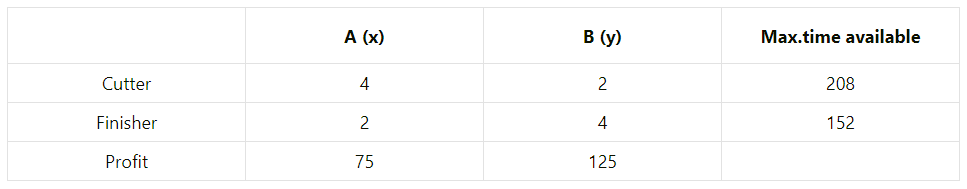Total time required for the cutter = 4x + 2y
Maximum available time 208 hours
∴ 4x+ 2y ≤ 208
Total time required for the finisher 2x +4y
Maximum available time 152 hours
2x + 4y ≤ 152
Total Profit is 75x + 125y
∴ L.P.P. of the above problem is
Minimize z = 75x + 125y
Subject to 4x+ 2y ≤ 208
2x + 4y ≤ 152
x ≥ 0; y ≥ 0
Graphical solution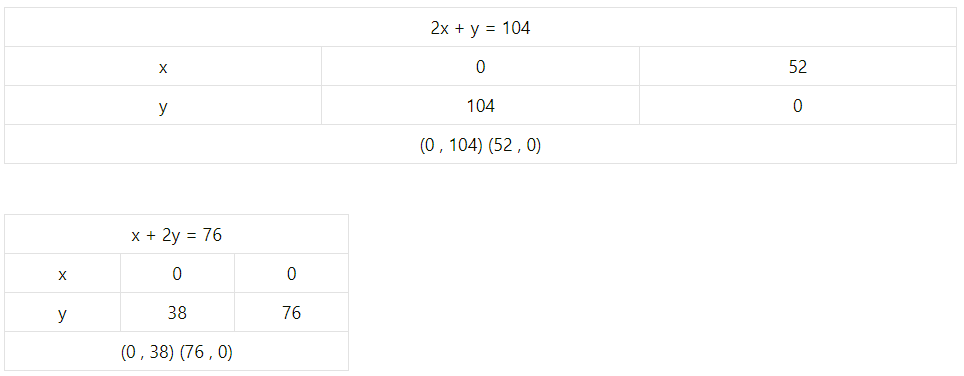Corner points
Now, Z at
x = (75x + 125y)
O(0, 0) = 75 × 0 + 125 × 0 = 0
A(52,0) = 75 × 52 + 125 × 0 = 3900
B(44, 16) = 75 × 44 + 125 × 16 = 5300
C(0, 38) = 75 × 0 + 125 × 38 = 4750
A person should make 44 items of type A and 16 Uems of type Band his returns are ₹ 5,300.

Question 13.
A firm manufactures two products A and B on which profit earned per unit ₹3/- and ₹4/- respectively. Each product is processed on two machines M1 and M2. The product A requires one minute of processing time on M1 and two minute of processing time on M2, B requires one minute of processing time on M1 and one minute of processing time on M2. Machine M1 is available for use for 450 minutes while M2 is available for 600 minutes during any working day. Find the number of units of product A and B to be manufactured to get the maximum profit.
Solution:
Let the firm manufactures x units of product
A and y units of product B.
The profit earned per unit of A is ₹3 and B is ₹ 4.
Hence, the total profit is z = ₹ (3x + 4y).
This is the linear function which is to be maximized.
Hence, it is the objective function.
The constraints are as per the following table :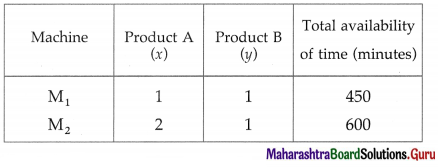From the table, the constraints are
x + y ≤ 450, 2x + y ≤ 600
Since, the number of gift items cannot be negative, x ≥ 0, y ≥ o.
∴ the mathematical formulation of LPP is,
Maximize z = 3x + 4y, subject to x + y ≤ 450, 2x + y ≤ 600, x ≥ 0, y ≥ 0.
Now, we draw the lines AB and CD whose equations are x + y = 450 and 2x + y — 600 respectively.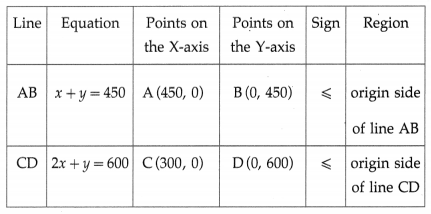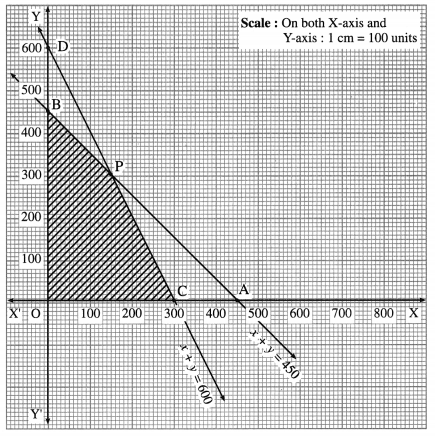The feasible region is OCPBO which is shaded in the graph.
The vertices of the feasible region are O(0, 0), C(300, 0), P and B (0, 450).
P is the point of intersection of the lines
2x + y = 600 … (1)
and x + y = 450 … (2)
On subtracting, we get
∴ x = 150
Substituting x = 150 in equation (2), we get
150 + y = 450
∴ y = 300
∴ P = (150, 300)
The values of the objective function z = 3x + 4y at these vertices are
z(O) = 3(0) + 4(0) = 0 + 0 = 0
z(C) = 3(300) + 4(0) = 900 + 0 = 900
z(P) = 3(150) + 4(300) = 450 + 1200 = 1650
z(B) = 3(0) + 4(450) = 0 + 1800 = 1800
∴ z has the maximum value 1800 when x = 0 and y = 450 Hence, the firm gets maximum profit of ₹ 1800 if it manufactures 450 units of product B and no unit product A.Question 14.
A firm manufacturing two types of electrical items A and B, can make a profit of ₹ 20/- per unit of A and ₹ 30/- per unit of B. Both A and B make use of two essential components a motor and a transformer. Each unit of A requires 3 motors and 2 transformers and each units of B requires 2 motors and 4 transformers. The total supply of components per month is restricted to 210 motors and 300 transformers. How many units of A and B should the manufacture per month to maximize profit? How much is the maximum profit?
Solution:
Let the firm manufactures x units of item A and y units of item B.
Firm can make profit of ₹ 20 per unit of A and ₹ 30 per unit of B.
Hence, the total profit is z = ₹ (20x + 30y).
This is the objective function which is to be maximized. The constraints are as per the following table :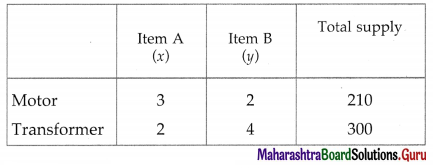From the table, the constraints are
3x + 2y ≤ 210, 2x + 4y ≤ 300
Since, number of items cannot be negative, x ≥ 0, y ≥ 0.
Hence, the mathematical formulation of given LPP is :
Maximize z = 20x + 30y, subject to 3x + 2y ≤ 210, 2x + 4y ≤ 300, x ≥ 0, y ≥ 0.
We draw the lines AB and CD whose equations are 3x + 2y = 210 and 2x + 4y = 300 respectively.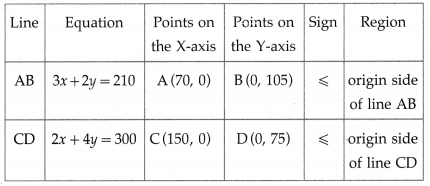The feasible region is OAPDO which is shaded in the graph.
The vertices of the feasible region are O (0, 0), A (70, 0), P and D (0, 75).
P is the point of intersection of the lines
2x + 4y = 300 … (1)
and 3x + 2y = 210 … (2)
Multiplying equation (2) by 2, we get
6x + 4y = 420
Subtracting equation (1) from this equation, we get
∴ 4x = 120 ∴ x = 30
Substituting x = 30 in (1), we get
2(30) + 4y = 300
∴ 4y = 240 ∴ y = 60
∴ P is (30, 60)
The values of the objective function z = 20x + 30y at these vertices are
z(O) = 20(0) + 30(0) = 0 + 0 = 0
z(A) = 20(70) + 30(0) = 1400 + 0 = 1400
z(P) = 20(30) + 30(60) = 600 + 1800 = 2400
z(D) = 20(0) + 30(75) = 0 + 2250 = 2250
∴ z has the maximum value 2400 when x = 30 and y = 60. Hence, the firm should manufactured 30 units of item A and 60 units of item B to get the maximum profit of ₹ 2400.